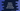# Python 3 Ordered Dictionary (OrderedDict) with example### Ordered Dictionary in python 3 with example :

Ordered Dictionary or OrderedDict is subclass of Dictionary in python . It acts similar to a dictionary, i.e. it will have all the methods than a dictionary have. Only difference is that it remembers the order how the keys are inserted to the dictionary. If you enter one value with a same key previously entered, it will remove the previous value and enter the new value at last. Let’s take a look into the difference between one OrderedDict and normal dictionary with an example :

### Python Example to show how OrderedDict works :

For one normal dictionary :

``````normal_dict = {}

normal_dict['1'] = "one"
normal_dict['2'] = "two"
normal_dict['3'] = "three"
normal_dict['4'] = "four"
normal_dict['5'] = "five"

print("Printing normal Dictionary : ")

for key,value in normal_dict.items():
print("key : {0},value : {1}".format(key,value))``````

The output is :

``````key : 1,value : one
key : 3,value : three
key : 2,value : two
key : 5,value : five
key : 4,value : four``````

So, the key,value pairs are not ordered as they are entered. Now, let’s take a look for the Ordered Dictionary :

``````import collections

ordered_dict = collections.OrderedDict()

ordered_dict['1'] = "one"
ordered_dict['2'] = "two"
ordered_dict['3'] = "three"
ordered_dict['4'] = "four"
ordered_dict['5'] = "five"

print("Printing Ordered Dictionary : ")

for key,value in ordered_dict.items():
print("key : {0},value : {1}".format(key,value))``````

The output is :

``````key : 1,value : one
key : 2,value : two
key : 3,value : three
key : 4,value : four
key : 5,value : five``````

The key,value pairs are printed same way they entered.

### Pop an item in Ordered Dict :

We can pop an item from an OrderedDict using popitem method. popitem(last=True) method is used to pop an item. The flag last is a boolean flag. If last is true, the pairs are returned in LIFO order. Else returned in FIFO order. Let’s try to understand this with an example :

``````import collections

ordered_dict = collections.OrderedDict()

ordered_dict['1'] = "one"
ordered_dict['2'] = "two"
ordered_dict['3'] = "three"
ordered_dict['4'] = "four"
ordered_dict['5'] = "five"

print("pop value with True flag {} ".format(ordered_dict.popitem(True)))
print("pop value with False flag {} ".format(ordered_dict.popitem(False)))``````

### Output :

``````pop value with True flag ('5', 'five')
pop value with False flag ('1', 'one')``````

### Comparing two Ordered Dictionary :

If we compare two normal dictionaries, it checks if all items are equal or not. For Ordered Dictionary, it also checks the order how the elements are added to both dictionaries. Let’s take a look :

### Example to compare two normal and ordered dictionaries :

``````import collections

normal_dict1 = {}
normal_dict2 = {}

normal_dict1['1'] = "one"
normal_dict1['2'] = "two"
normal_dict1['3'] = "three"
normal_dict1['4'] = "four"
normal_dict1['5'] = "five"

normal_dict2['2'] = "two"
normal_dict2['1'] = "one"
normal_dict2['4'] = "four"
normal_dict2['3'] = "three"
normal_dict2['5'] = "five"

ordered_dict1 = collections.OrderedDict()
ordered_dict2 = collections.OrderedDict()

ordered_dict1['1'] = "one"
ordered_dict1['2'] = "two"
ordered_dict1['3'] = "three"
ordered_dict1['4'] = "four"
ordered_dict1['5'] = "five"

ordered_dict2['2'] = "two"
ordered_dict2['1'] = "one"
ordered_dict2['4'] = "four"
ordered_dict2['3'] = "three"
ordered_dict2['5'] = "five"

if normal_dict1 == normal_dict2 :
print("Normal dictionaries are equal.")
else :
print("Normal dictionaries are not equal.")

if ordered_dict1 == ordered_dict2 :
print("Ordered dictionaries are equal.")
else :
print("Ordered dictionaries are not equal.")``````

### Output :

``````Normal dictionaries are equal.
Ordered dictionaries are not equal.``````

So, the order is equally important for Ordered dictionaries if you are comparing two.